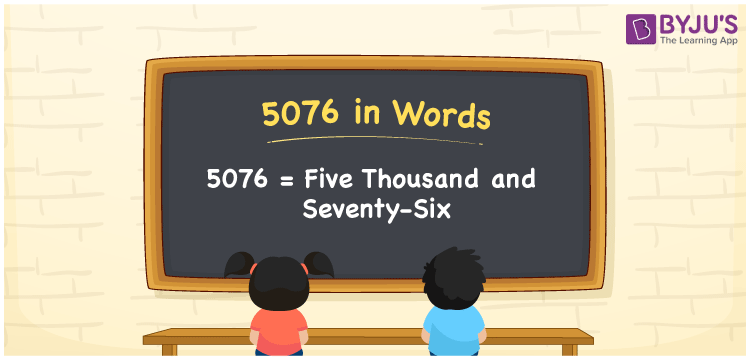# 5076 in Words

We can write 5076 in words as Five thousand and seventy-six. Suppose you booked a Bluetooth speaker for Rs. 5076, then you can say, “I booked a Bluetooth speaker for Five thousand and seventy-six rupees”. Thus, Five thousand and seventy-six is the word form of the cardinal number 5076. Let’s learn how to write the number 5076 in words using a place value chart here in this article.

 5076 in words Five thousand and seventy-six Five thousand and seventy-six in Numbers 5076

## 5076 in English Words

We generally write numbers in words using the English alphabet. Thus, we can spell 5076 in English as “Five thousand and seventy-six”.## How to Write 5076 in Words?

The number 5076 has four digits so to convert this number to words, we need to make a place value chart with four columns. This can be done as follows.

 Thousands Hundreds Tens Ones 5 0 7 6

Here, ones = 6, tens = 7, hundreds = 0, and thousands = 5.

These numbers can be written as follows.

5 × Thousand + 0 × Hundred + 7 × Ten + 6 × One

= 5 × 1000 + 0 × 100 + 7 × 10 + 6 × 1

= 5000 + 70 + 6

= Five thousand + Seventy + Six

= Five thousand and seventy-six

Therefore, 5076 in words = Five thousand and seventy-six.

5076 is a natural number that precedes 5077 and succeeds 5075.

5076 in words – Five thousand and seventy-six

Is 5076 an odd number? – No

Is 5076 an even number? – Yes

Is 5076 a prime number? – No

Is 5076 a composite number? – Yes

Is 5076 a perfect square number? – No

Is 5076 a perfect cube number? – No

## Frequently Asked Questions on 5076 in Words

Q1

### Write the number 5076 in words.

The number 5076 can be written in words as Five thousand and seventy-six.
Q2

### How do you write Rs. 5076 in words on a cheque?

On a cheque, we can write an amount of Rs. 5076 in words as “Five thousand and seventy-six rupees only.
Q3

### What is the number name of 5076?

The number name of 5076 is Five thousand and seventy-six.